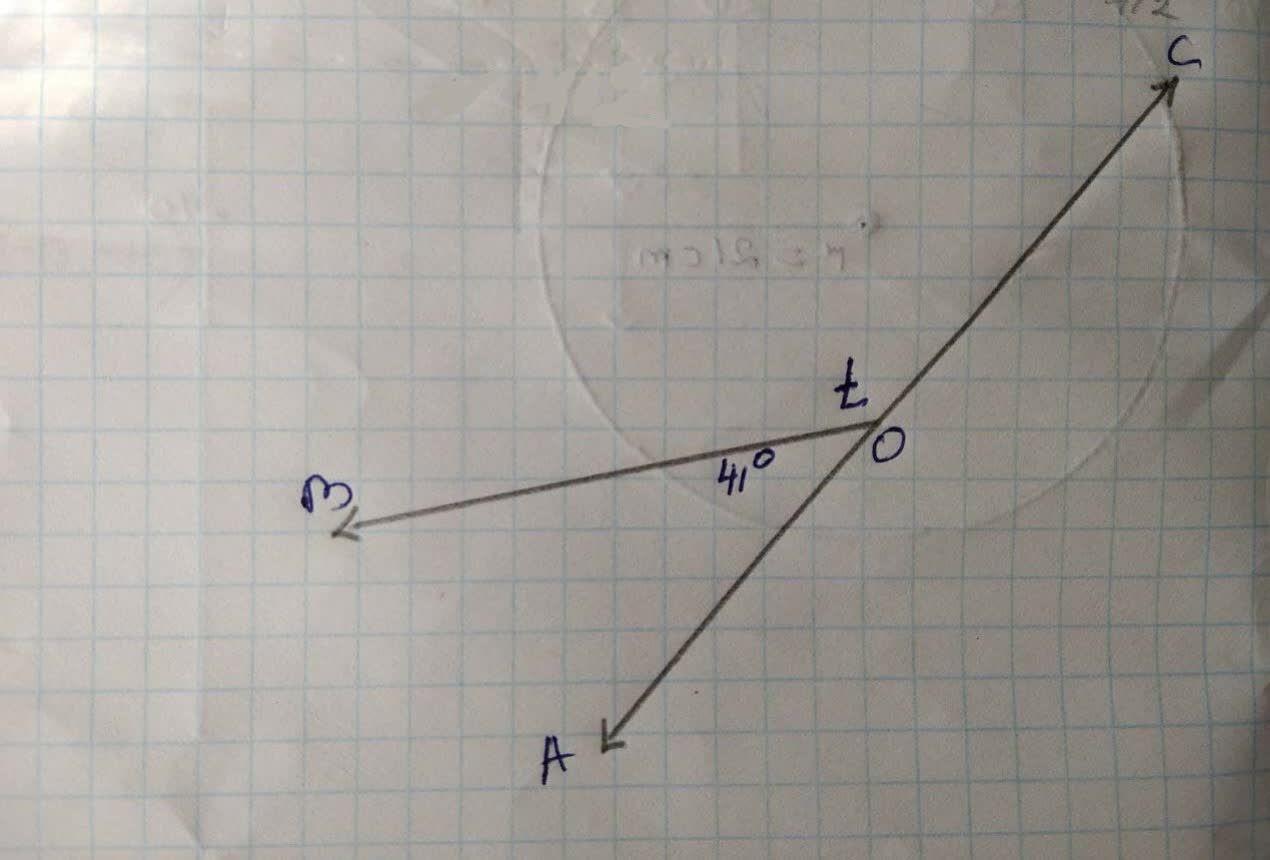Jason Watson

2021-11-28

What is the value of t?Melinda Olson

Given figurc
We find thc volue of t
From thc figure we sec thc t
$\mathrm{\angle }AOB+\mathrm{\angle }BOC=180°$

$⇒41°+t=180°$
$\left[\therefore \mathrm{\angle }AOB=41°\mathrm{\angle }BOC=t\right]$
$⇒t=180°-41°$
$⇒t=139°$
Hence $t=139°$Jeffrey Jordon